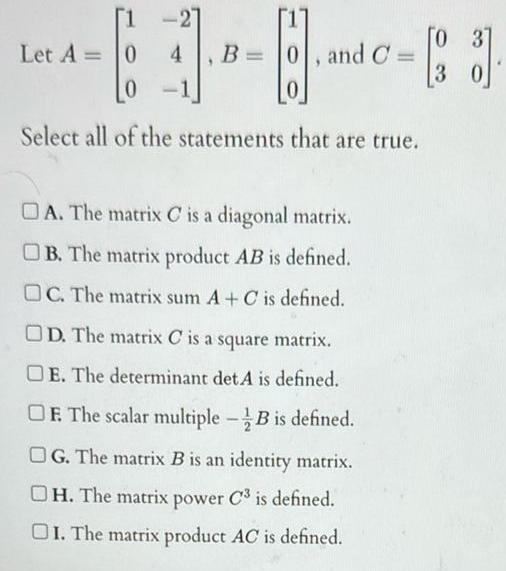Question:

# 1 Let A 0 0 Select all of the statements that are true B 0

Last updated: 11/13/20231 Let A 0 0 Select all of the statements that are true B 0 and C 0 4 OA The matrix Cis a diagonal matrix B The matrix product AB is defined OC The matrix sum A C is defined OD The matrix Cis a square matrix OE The determinant det A is defined OF The scalar multiple B is defined OG The matrix B is an identity matrix OH The matrix power C is defined OI The matrix product AC is defined 0 83 3 0